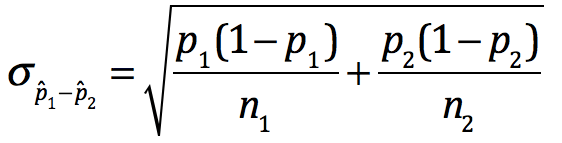## Day 56 - Lesson 6.2

##### Learning Targets
• Calculate the mean and standard deviation of the sum or difference of random variables.

• Find probabilities involving the sum or difference of independent Normal random variables.

##### Activity:

This Activity continues with the context of “hourly wage”. This time, we have decided to boost the hourly wage (X) for each student with a raise, according to a random variable (Y). They must calculate the probability distribution of their new wage N = X + Y. Students will be thrilled about their raise and will be very likely to work harder today in class.

##### Probability and Independence

Students must first consider all the possible values for the new wage N. It is possible that they will get assigned an hourly wage X = \$9 and a raise Y = \$1, giving them a new wage N = 9 + 1 = \$10. To find the probability of this new wage, we must go back to the General Multiplication Rule from Chapter 5.

Important: To use this General Multiplication Rule to create the probability distribution for N, we must assume the two random variables are independent. This helps explain why your textbook says that random variables must be independent in order to variances.

##### One Does Not Simply Add Standard Deviations

As students finish up this activity, challenge them to think about the formulas for calculating the mean and standard deviation when combining random variables. They will likely realize that the means and variances add. Most important, they realize:Now any time that students make this error throughout the rest of the course, we just make this hand gesture and they get it.

##### What About Subtracting Random Variables?

In the debrief, we asked students how this activity would change if instead of a raise, we had imposed an hourly pay cut. The probability distribution of N would have all the same probabilities, just some new values for N.

We had students put this distribution in their calculators and find the mean, variance, and standard deviation. They realized that the means subtract (as expected), and the variances add (not expected). To help them understand that the variability is increasing, take a simple look at range, which increases from 6 for X to 8 for N.

##### Why is This Important for AP Stats?

Later in Chapter 10, students will need the formulas for the standard deviation of the sampling distribution for the difference in proportions and the standard deviation of the sampling distribution for the difference in means (which requires them to add variances and square root!)